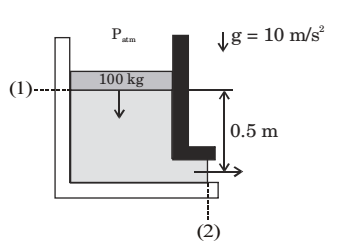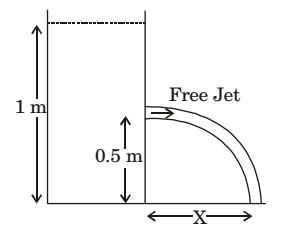## Fluid Mechanics and Hydraulic Machinery Miscellaneous

#### Fluid Mechanics and Hydraulic Machinery

1. The necessary and sufficient condition which brings about separation of boundary layer is
 dp > 0 (True / False) dx

1. NA

##### Correct Option: A

NA

1. The predominant forces acting on an element of fluid in the boundary layer over a flat plate in a uniform parallel stream are

1. NA

##### Correct Option: B

NA

1. The instantaneous stream wise velocity of a turbulent flow is given as follows: u(x, y, z, t) = u(x, y, z) + u'(x, y, z, t) The time average of the fluctuating velocity u (x, y, z, t) is

1. Time average of fluctuating velocity is zero.

##### Correct Option: C

Time average of fluctuating velocity is zero.

1. A frictionless circular piston of area 10-2m2 and mass 100 kg sinks into a cylindrical container of the same area filled with water of density 1000 kg/m3 as shown in the figure. The container has a hole of area 10–3m2 at the bottom that is open to the atmosphere. Assuming there is no leakage from the edges of the piston and considering water to be incompressible, the magnitude of the piston velocity (in m/s) at the instant shown is _________ (correct to three decimal places).1. A1 V1 = A2 V2

##### Correct Option: A

A1 V1 = A2 V2

1. A tank open at the top with a water level of 1 m, as shown in the figure, has a hole at a height of 0.5 m. A free jet leaves horizontally from the smooth hole. The distance X(in m) where the jet strikes the floor is1. ux = √2gh= √2 × 9.8 × 0.5
ux = 3.13 m/s
Now for distance x
we require ux so–

 x = ux . t + 1 × a × b 2

x = 3.13 t
(∵ acceleration (a) = 0 in horizontal direction)
 Now for t ⇒ y = uyt + 1 gt² 2

 ⇒ 0.5 = 0 + 1 × 9.81 × t² [∵ uy = 0] 2

t = 0.319 sec.
so distance, x = 3.13 × 319 = 1m

##### Correct Option: B

ux = √2gh= √2 × 9.8 × 0.5
ux = 3.13 m/s
Now for distance x
we require ux so–

 x = ux . t + 1 × a × b 2

x = 3.13 t
(∵ acceleration (a) = 0 in horizontal direction)
 Now for t ⇒ y = uyt + 1 gt² 2

 ⇒ 0.5 = 0 + 1 × 9.81 × t² [∵ uy = 0] 2

t = 0.319 sec.
so distance, x = 3.13 × 319 = 1m